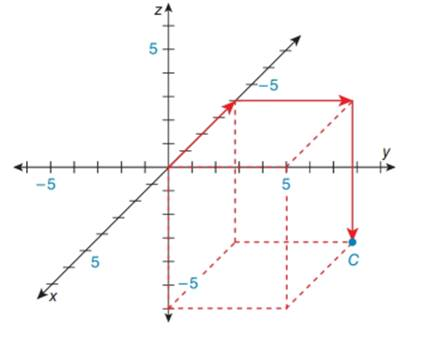Chapter 10.6, Problem 2E### Elementary Geometry for College St...

6th Edition
Daniel C. Alexander + 1 other
ISBN: 9781285195698

#### Solutions

Chapter
Section### Elementary Geometry for College St...

6th Edition
Daniel C. Alexander + 1 other
ISBN: 9781285195698
Textbook Problem
1 views

# In the Cartesian coordinate system below, name the ordered triple x ,   y ,   z represented by point C . Also, plot the point D 5 ,   - 4 ,   6 .To determine

To find:

The name of the ordered triple found in the given figure and plo the point C5, -4, 6.

Explanation

The given figure is shown below.

In a three dimensional Cartesian coordinate system, x-axis moves forward and backward.

Forward means positive coordinate.

Backward means negative coordinate.

y-axis moves right and left.

Right means positive coordinate.

Left means negative coordinate.

z-axis moves upward and downward.

Upward means positive coordinate.

Downward means negative coordinate.

In the given figure,

Beginning from the origin, x-axis moves 4 units backward, y-axis moves 5 units to the right and z-axis moves 6 units downwards to arrive at the given point A.

Hence, the ordered triple is C-4, 5, 6

### Still sussing out bartleby?

Check out a sample textbook solution.

See a sample solution

#### The Solution to Your Study Problems

Bartleby provides explanations to thousands of textbook problems written by our experts, many with advanced degrees!

Get Started

#### Find an equation of the vertical line that passes through (0, 5).

Applied Calculus for the Managerial, Life, and Social Sciences: A Brief Approach

Mathematics For Machine Technology

#### Polar coordinates of the point with rectangular coordinates (5, 5) are: (25, 0)

Study Guide for Stewart's Single Variable Calculus: Early Transcendentals, 8th

#### For and

Study Guide for Stewart's Multivariable Calculus, 8th